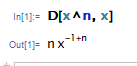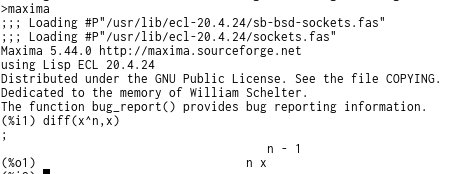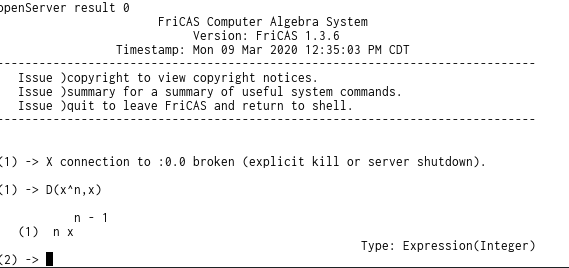## 5961 Reputation

9 years, 140 days

## MaplePrimes Activity

### These are answers submitted by nm

Alternatively, a way to factorizeintocould do the trick.

```restart;
expr:=a*x+6*x^2-10;
tmp:=select(has,expr,x);
factor(tmp)+expr-tmp;
```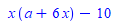I am not sure if is possible to write Q3 = gamma*Q1 + (1-gamma)*Q2

Maple says it is not possible. You can try simplify with side relations

```restart;
Q3 := 1/(v)*(    v*pi-  (   (1-alpha*gamma) *pi * r  - (1-pi)*B*alpha  + (gamma*pi + (1-pi)*h)    )   );
eqs:={ 1/v *(  v*pi    -  (   h - (1-pi)*B   )      )=Q2,1/v*(   v*pi     - (   pi*r+ (1-pi)*h  )   )=Q1};
simplify(Q3,eqs);```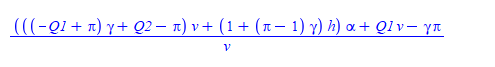I have not used this command before myself in Maple, but may this is what you want

```plots:-conformal(exp(2*z), z=0..1+I);
```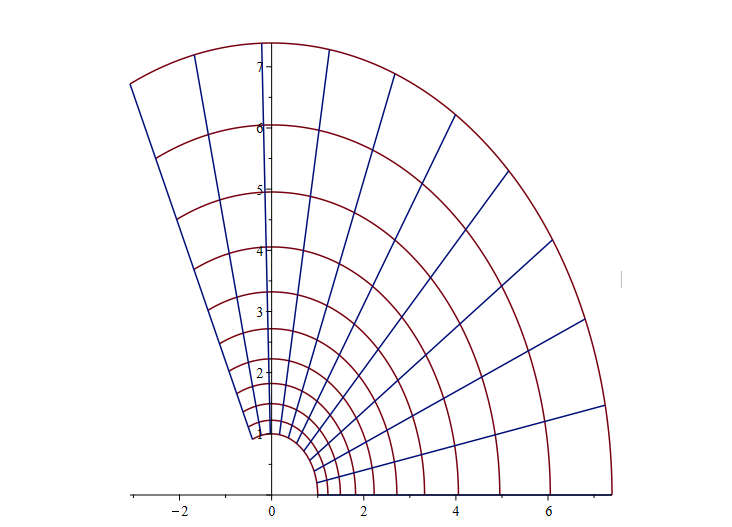may be you can use the definition of concave function. From Wiki

differentiable function f is (strictly) concave on an interval if and only if its derivative function f ′ is (strictly) monotonically decreasing on that interval, that is, a concave function has a non-increasing (decreasing) slope.

So just need one counter example. This is your g(x) function which is increasing and concave function g:=x-exp(-x).

If you plot the derivative of   (2*x+5)*g(x), you will see is not concave.

Why would you load LinearAlgebra and then at same time make call to linalg:- ?

Important: The linalg package has been deprecated. Use the superseding packages, LinearAlgebra

if you remove that then it works. It might have to do with how linalg works. I do dot know for sure, but this works.

To multiply these matrices/vectors, just use the dot. So intead of what you had

linalg:-multiply(Ts_sksul, XCin)

you can just do

Ts_sksul.XCin

fixed.mw

diff(x^n,x) does not equal n*x^(n-1), but rather 0 if n=1

you probably meant rather 1, and not rather 0..

This seems like a bug to me

it is not a bug. all CAS systems work on a general expressions, not specific values.